# 8.1 Algebraic Curves

Curves that can be given in implicit form as f(x,y)=0, where f is a polynomial, are called algebraic. The degree of f is called the degree or order of the curve. Thus conics (Section 7) are algebraic curves of degree two. Curves of degree three already have a great variety of shapes, and only a few common ones will be given here.

The simplest case is when the curve is the graph of a polynomial of degree three: y=ax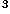+bx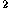+cx+d, with a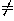0. This curve is a (general) cubic parabola (Figure 1). It is symmetric with respect to the point B where x=-b/3a.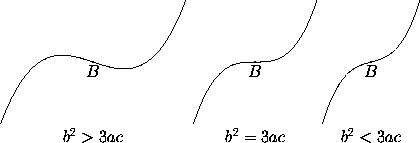Figure 1: The general cubic parabola for a>0. For a<0, reflect in a horizontal line.

The semicubic parabola (Figure 2, left) has equation y=kx; by proportional scaling one can take k=1.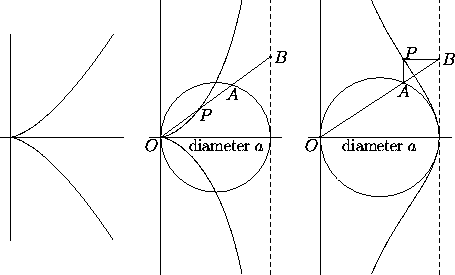Figure 2: The semicubic parabola, the cissoid of Diocles, and the witch of Agnesi

This curve should not be confused with the cissoid of Diocles (Figure 2, middle), which has equation (a-x)y=xwith a0. The latter is asymptotic to the line x=a, while the semicubic parabola has no asymptotes. The cissoid's points are characterized by the equality OP=AB in Figure 2, right. One can take a=1 by proportional scaling.

More generally, any curve of degree three with equation (x-x)y=f(x), where f is a polynomial, is symmetric with respect to the x-axis and asymptotic to the line x=x. In addition to the cissoid, the following particular cases are important:

• The witch of Agnesi has equation xy=a(a-x), with a0, and is characterized by the geometric property shown in Figure 2, right. The same property provides the parametric representation x=a(1+sin 2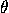), y=a tan. Once more, proportional scaling reduces to the case a=1.
• The folium of Descartes (Figure 3, left) has equation (x-a)y=-x(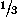x+a), with a0 (reducible to a=1 by proportional scaling). By rotating 135°(right) we get the alternative and more familiar equation x+y=cxy, where c=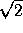a. The folium of Descartes is a rational curve, this is, it has a parametric representation by rational functions. In the tilted position such a representation is x=ct/(1+t), y=ct/(1+t) (so that t=y/x).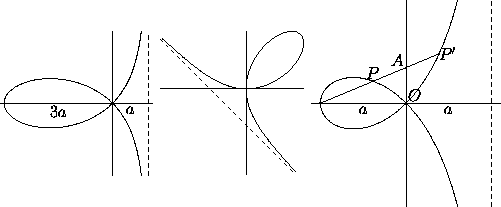Figure 3: The folium of Descartes in two positions, and the strophoid.

• The strophoid has equation (x-a)y=-x(x+a), with a0 (reducible to a=1 by proportional scaling). It satisfies the property AP=AP'=OA in Figure 3, right; this means that POP' is a right angle. The strophoid has the polar representation r=-a cos 2sec, and the rational parametric representation x=a(t-1)/(t+1), y=at(t-1)/(t+1) (so that t=y/x).

Among the important curves of degree four are the following:

• A Cassini's oval is characterized by the following condition: given two foci F and F', a distance 2a apart, a point P belongs to the curve if the product of the distances PF and PF' is a constant k. If the foci are on the x-axis and equidistant from the origin, the curve's equation is (x+y+a)-4ax=k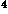. Changes in a correspond to rescaling, while the value of k/a controls the shape: the curve has one smooth piece, one piece with a self-intersection, or two pieces depending on whether k is greater than, equal to, or smaller than a (Figure 4). The case k=a is also known as the lemniscate (of Jakob Bernoulli); the equation reduces to (x+y)=a(x-y), and upon a 45° rotation to (x+y)=2axy. Each Cassini's oval is the section of a torus of revolution by a plane parallel to the axis of revolution.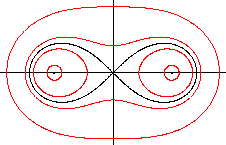Figure 4: Cassini's ovals for k=.5a, .9a, 1.1a and 1.5a (from the inside to the outside). The foci (dots) are at x=a and x=-a. The black curve, k=a, is also called Bernoulli's lemniscate.

• A conchoid of Nichomedes is the set of points such that the signed distance AP in Figure 5, left, equals a fixed real number k (the line L and the origin O being fixed). If L is the line x=a, the conchoid has polar equation

r=a sec+k.

Once more, a is a scaling parameter, and the value of k/a controls the shape: when k>-a the curve is smooth, when k=-a there is a cusp, and when k<-a there is a self-intersection. The curves for k and -k can also be considered two leaves of the same conchoid, with cartesian equation (x-a)(x+y)=kx.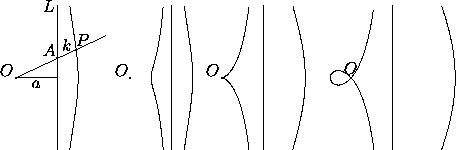Figure 5: Defining property of the conchoid of Nichomedes (left), and curves for k=±.5a, ka, and k=±1.5a (right).

• A limaçon of Pascal is the set of points such that the distance AP in Figure 6, left, equals a fixed positive number k measured on either side (the circle C and the origin O being fixed). If C has diameter a and center at (0,½a), the limaçon has polar equation

r=a cos+ k,

and cartesian equation (x+y-ax)=k(x+y). The value of k/a controls the shape, and there are two particularly interesting cases. For k=a we get a cardioid (see also Figure 8.2.2 , right). For ak we get a curve that can be used to trisect an arbitrary angle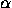: if we draw a line L through the center of the circle C and making an anglewith the positive x-axis, and if we call P the intersection of L with the limaçon a=2k, the line from O to P makes an anglewith L.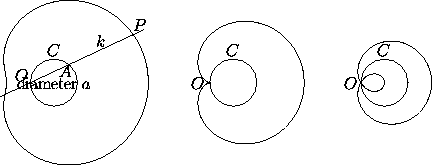Figure 6: Defining property of the limaçon of Pascal (left), and curves for k=1.5a, k=a, and k=.5a (right). The middle curve is the cardioid, the one on the right a trisectrix.

Hypocycloids and epicycloids with rational ratio (see next section) are also algebraic curves, generally of higher degree.

Next: 8.2 Roulettes (Spirograph Curves)
Up: 8 Special Plane Curves
Previous: 8 Special Plane CurvesThe Geometry Center Home Page

Silvio Levy
Wed Oct 4 16:41:25 PDT 1995

This document is excerpted from the 30th Edition of the CRC Standard Mathematical Tables and Formulas (CRC Press). Unauthorized duplication is forbidden.